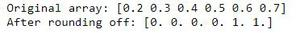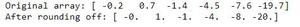How to round elements of the NumPy array to the nearest integer?

• Last Updated : 29 Aug, 2020

Prerequisites: Python NumPy

In this article, let’s discuss how to round elements of the NumPy array to the nearest integer. numpy.rint() function of Python that can convert the elements of an array to the nearest integer.

Syntax: numpy.rint(x, /, out=None, *, where=True, casting=’same_kind’, order=’K’, dtype=None, subok=True[, signature, extobj]) = <ufunc ‘rint’>

Example 1:

Python3

 import numpy as n  # create arrayy = n.array([0.2, 0.3, 0.4, 0.5, 0.6, 0.7])print("Original array:", end=" ")print(y)  # rount to nearest integery = n.rint(y)print("After rounding off:", end=" ")print(y)

Output:Example 2:

Python3

 import numpy as n  # create arrayy = n.array([-0.2, 0.7, -1.4, -4.5, -7.6, -19.7])print("Original array:", end=" ")print(y)  # rount to nearest integery = n.rint(y)print("After rounding off:", end=" ")print(y)

Output:My Personal Notes arrow_drop_up